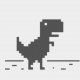# offset table (intersection points)

## Recommended Postshi all,

i have drawings that contains stations and waterlines as polyline or spline.

(in att. dwg named as ST0, ST1...ST20, and WL0 to WL8)

there may be other stations or waterlines such as ST0.5, ST1.5, WL9, WL10...

i need a table that shows distance of intersection points from CL. (see att. dwg)

is it possible to make this table by lisp?

lines.dwg

##### Share on other sitesSuggestion: make all layers unique for each (section) lwpolyline, i.e: "ST0" "ST1" "ST10" etc..

otherwise we can't populate the intersection points if all sections are in same layer "ST17" ?!

```(vl-load-come)

[color="green"];Rev 1[/color]
(defun c:[b]test1[/b] (/ *error* p ss lst fn f  [color="red"]hp:table[/color] ) [color="green"];<-- localize=output csv, global=output table, i.e: To remove [color="red"]hp:table[/color] from variable list[/color]
;hanhphuc 15.05.2018
(defun *error* (msg)
(if	f
(close f)
)
)

(if (and
(setq p (getpoint "\nSpecify base point.. "))
(setq ss (ssget '((0 . "LWPOLYLINE") (8 . "[color="purple"][b]GRID,*ST*[/b][/color]"))))
(setq lst (mapcar ''((x)
(setq obj (vlax-ename->vla-object x))
(cons (vla-get-Layer obj)
(reverse (mapcar ''((a / ip)
(if
(setq ip (vlax-invoke obj 'intersectwith (vlax-ename->vla-object a) AcExtendNone))
(rtos (* (-(car (trans ip 0 1))(car p)) 1e-3) 2 3)
"-"
)
)
(vl-remove-if ''((x)(or (apply 'equal (append (mapcar ''((f) (car (trans ( f x ) 0 1 )))
(list vlax-curve-getstartpoint vlax-curve-getEndpoint)) '(1e-3))
)
(not (wcmatch (cdr(assoc 8 (entget x))) [b][color="purple"]"GRID"[/color][/b]) )
)
) (acet-ss-to-list ss))
)
)
)
)
(vl-remove-if-not ''((x)(wcmatch (cdr(assoc 8 (entget x))) [color="purple"][b]"*ST*"[/b][/color] )) (acet-ss-to-list ss))
)
)
(setq lst (cons '([b][color="purple"]"SECTIONS" "WL0.0" "WL0.5" "WL1.0" "WL2.0" "WL3.0" "WL4.0" "WL5.0" "WL6.0" "WL7.0" "WL8.0"[/color][/b])
(vl-sort lst
''((a b) (apply '< (mapcar ''((x) (car ([color="blue"]LM:parsenumbers[/color] x))) (list (car a) (car b)))))) )
)

); and
(if (and hp:table (setq p (getpoint "\nSpecify insertion point.. ")))
(hp:table lst (trans p 1 0) "OFFSET TABLE" (getvar 'textsize))
(progn
(and (setq fn (vl-filename-mktemp "tmp" (getvar 'dwgprefix) ".csv"))
(setq f (open fn "w"))
(foreach x lst (write-line (apply 'strcat (mapcar ''((a) (strcat a ",")) x)) f))
(progn (if	f (close f) )
(vl-cmdf "start" fn)
)
)
)
); if

(princ "\nNothing!")

)
(princ)
) ;_ end of defun

[color="green"]
;; Parse Numbers  -  Lee Mac
;; Parses a list of numerical values from a supplied string.[/color]

(defun LM:parsenumbers ( str )
(   (lambda ( l )
(strcat "("
(vl-list->string
(mapcar
'(lambda ( a b c )
(if (or (< 47 b 58)
(and (= 45 b) (< 47 c 58) (not (< 47 a 58)))
(and (= 46 b) (< 47 a 58) (< 47 c 58))
)
b 32
)
)
(cons nil l) l (append (cdr l) '(()))
)
)
")"
)
)
)
(vl-string->list str)
)
)
```

This routine is only a test on drawing you provided,

i.e. you need to modify the TEXTSIZE to suit your drawing

Command: TEXTSIZE

then edit new value 200.0

or add (setvar 'textsize 200.) as default in the code

Edited by hanhphuc
rev1, allow pick base point

##### Share on other siteshi hanhphuc,

thnx for great code and suggestion. really fast job.

but i think something missing about "hp:table" that i dont have.

so the code gives me only temp001.csv file.

can u fix this?

##### Share on other sitesis there another way to make autocad table from the csv file?

##### Share on other siteshi hanhphuc,

thnx for great code and suggestion. really fast job.

but i think something missing about "hp:table" that i dont have.

so the code gives me only temp001.csv file.

can u fix this?

hi, actually you can direct copy from .csv then paste as OLE object in ACAD.

This forum as we are volunteers , we prefer OP attempt to code & solve it, sometimes busy may overlook.

it was external global function omitted, so should be unique name

```(defun hp:TABLE ( lst pt str sz / obj r i )
;hanhphuc

[color="green"];RetVal = object.AddTable(InsertionPoint, NumRows, NumColumns, RowHeight, ColWidth)  [/color]

(setq
obj
(vlax-invoke
((lambda (doc)
(foreach x '(ActiveDocument ActiveLayout Block) (setq doc (vlax-get doc x)))
)
)
pt 			; InsertionPoint
(if str
(+ 1 (length lst)) ; NumRows
(length lst)
)
(length (car lst)) 	; NumColumns
(* sz 3.0) 		; RowHeight
(* sz 10.)		; ColWidth
)
)

(foreach x (list acTitleRow acHeaderRow acDataRow)
(vla-settextheight obj x sz)
(vla-settextStyle obj x (getvar 'textStyle))
)

(setq r	(if str
(progn (vla-settext obj 0 0 str) 1 ) 0
)
)
(foreach x lst
(setq i 0)
(foreach \$ x
(vla-setcellalignment obj r i acMiddleCenter)
(vla-settext obj r i \$)
(setq i (1+ i))
) ;_ end of foreach
(setq r (1+ r))
) ;_ end of foreach
(vla-put-regeneratetablesuppressed obj :vlax-false)
obj
) ;_ end of defun

```

Here's another workaround

1.pick base point at zero axis

2.select crossing or window

3.Insert table##### Share on other sitesthank you very much hanhphuc, this is really great## Join the conversation

You can post now and register later. If you have an account, sign in now to post with your account.
Note: Your post will require moderator approval before it will be visible.×   Pasted as rich text.   Paste as plain text instead

Only 75 emoji are allowed.